#0
0
01. 云栖社区>
2. CDA数据分析师>
3. 博客>
4. 正文

## 手把手教你用Python分析豆瓣电影——以《我不是药神》《邪不压正》为例

《我不是药神》是由文牧野执导，宁浩、徐峥共同监制的剧情片，徐峥、周一围、王传君、谭卓、章宇、杨新鸣等主演 。影片讲述了神油店老板程勇从一个交不起房租的男性保健品商贩程勇，一跃成为印度仿制药“格列宁”独家代理商的故事。

0.需求分析

1.前期准备

1.1 网页分析

tips发现

1.2 页面布局分析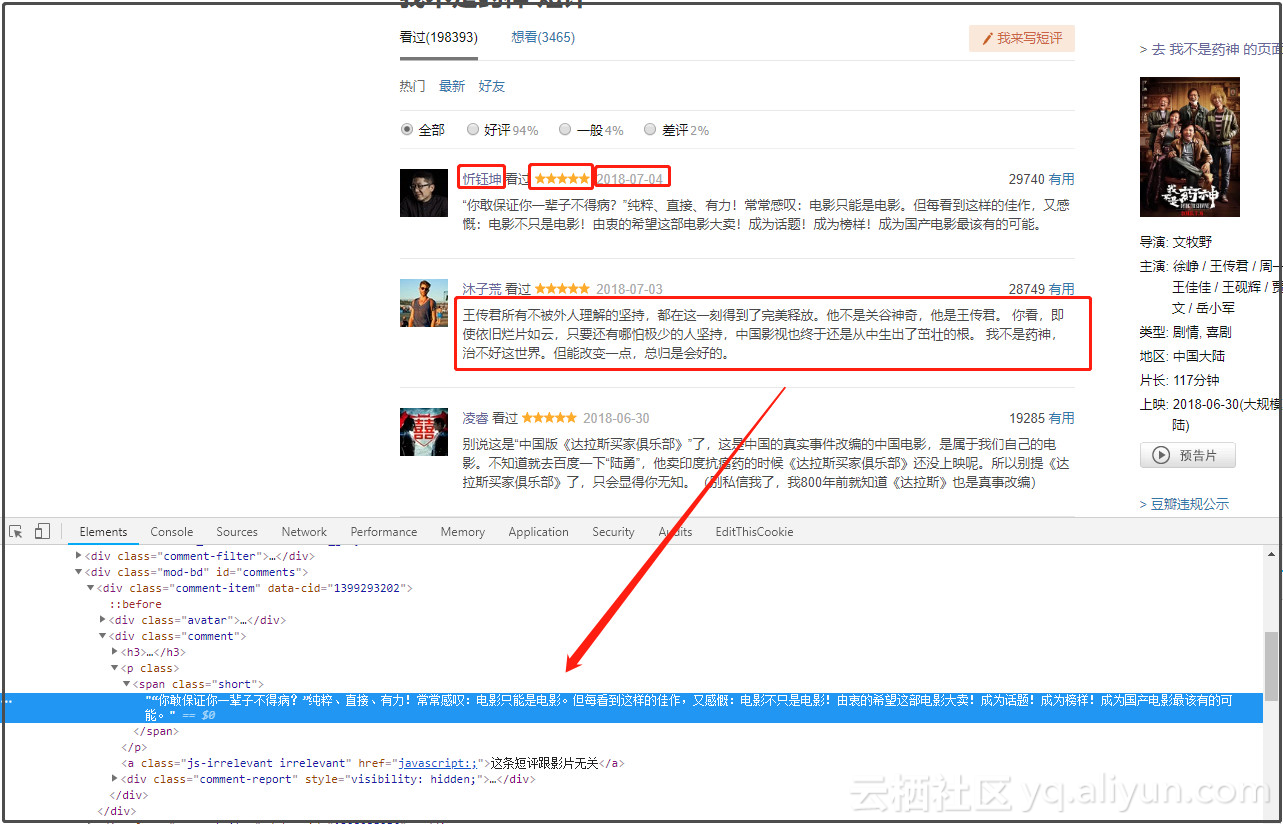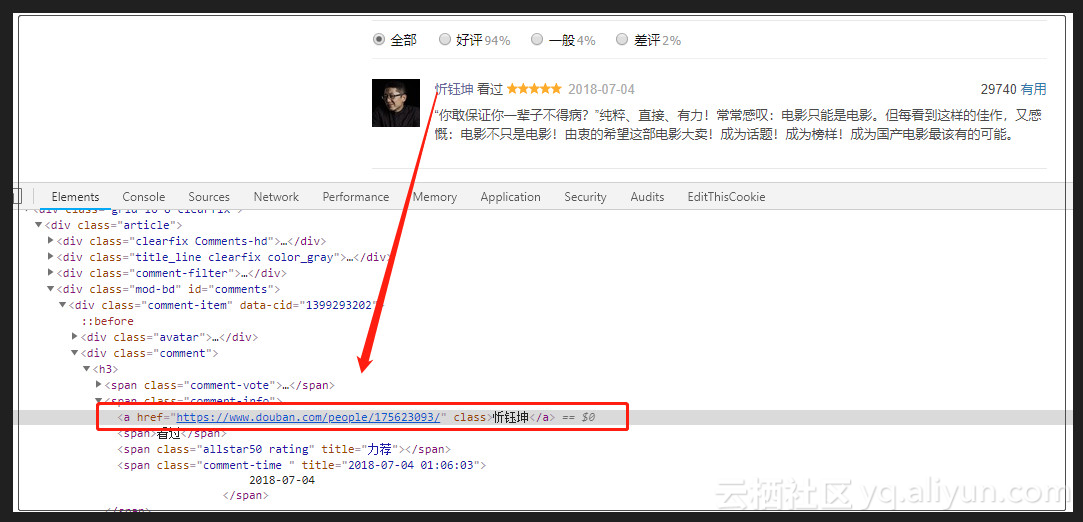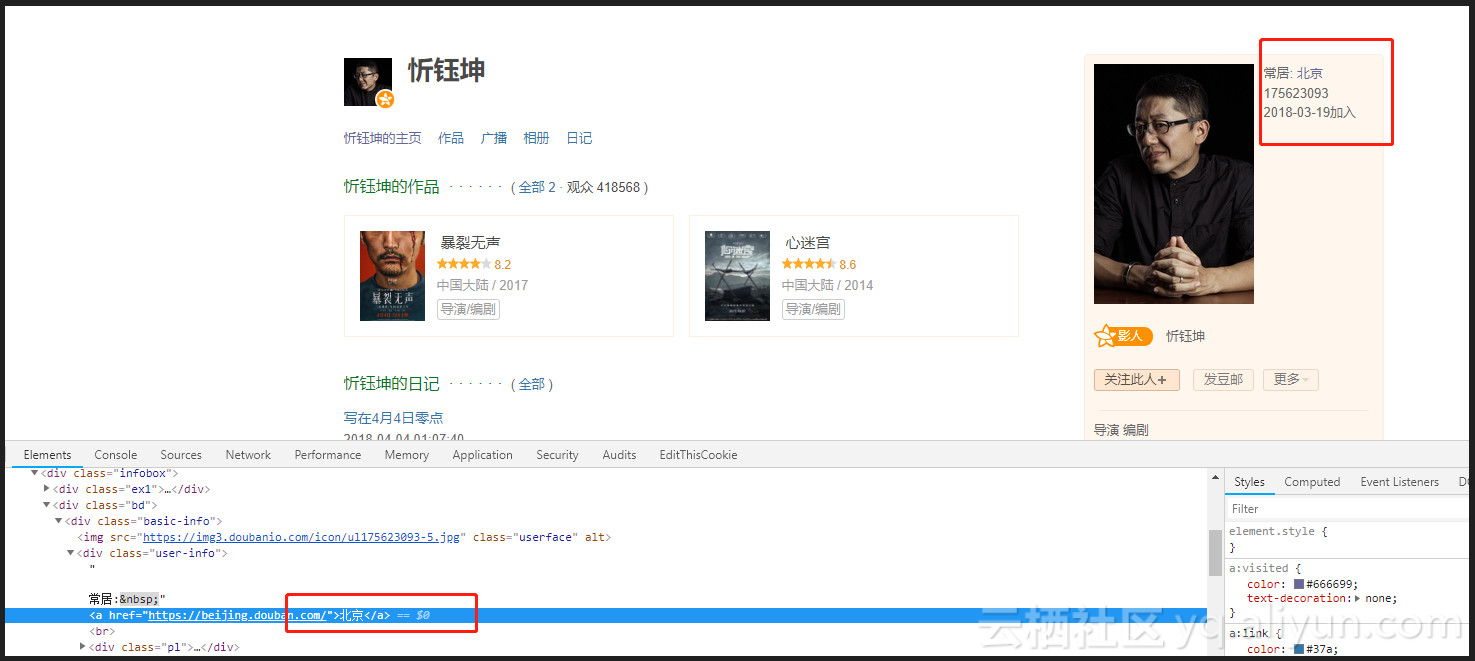2.数据获取-爬虫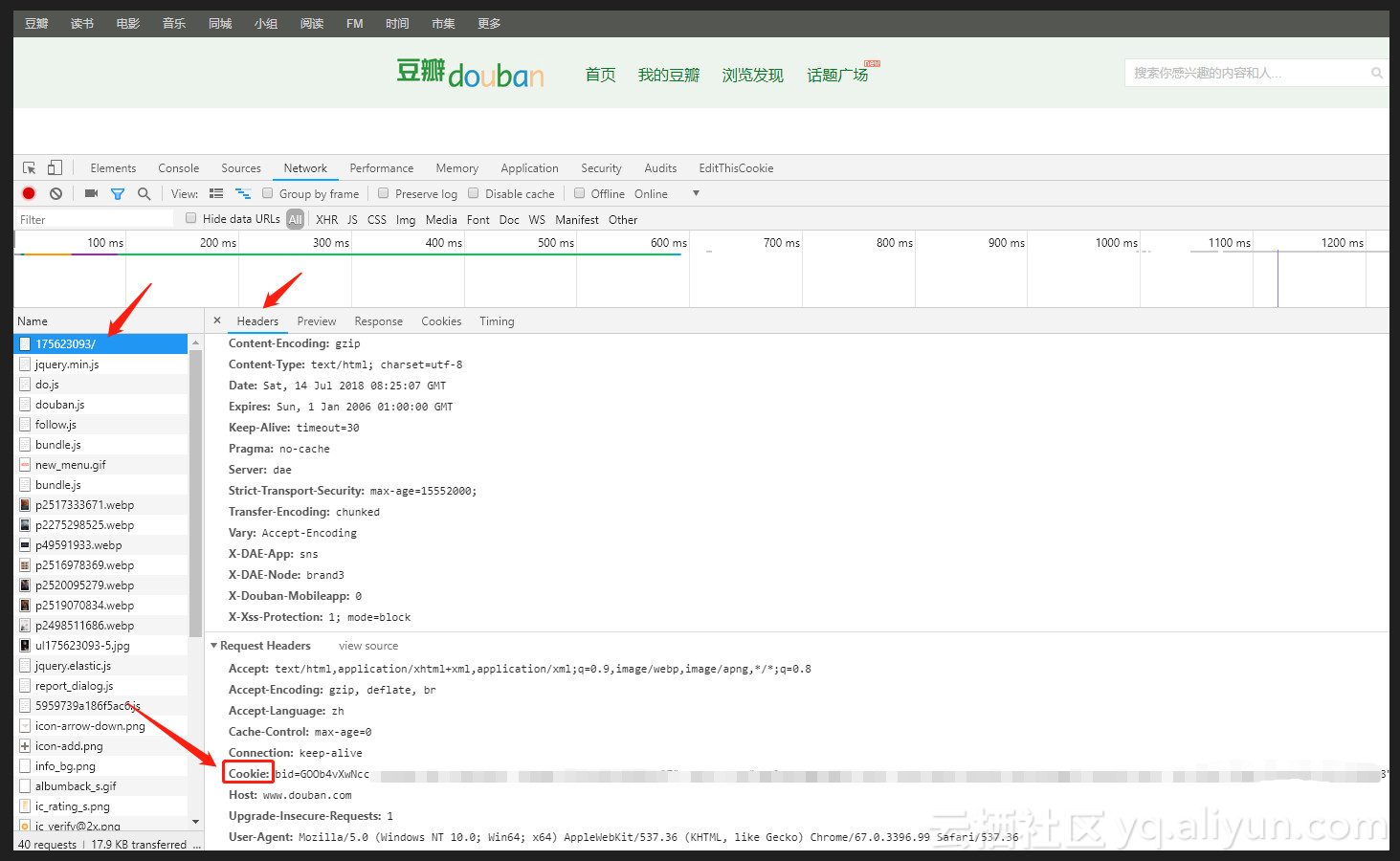headers = {
'User-Agent': 'Mozilla/5.0 (Windows NT 10.0; Win64; x64) AppleWebKit/537.36 (KHTML, like Gecko) Chrome/67.0.3396.99 Safari/537.36'}
"push_noty_num=0; push_doumail_num=0; ap=1; loc-last-index-location-id="108288"; ll="108288"; dbcl2="187285881:N/y1wyPpmA8"; ck=4wlL'}
url = "https://movie.douban.com/subject/" + str(id) + "/comments?start=" + str(page * 10) + "&limit=20&sort=new_score&status=P"
res.encoding = "utf-8"
if (res.status_code == 200):
print("\n第{}页短评爬取成功！".format(page + 1))
print(url)
else:
print("\n第{}页爬取失败！".format(page + 1))


2.3延时反爬虫

time.sleep(round(random.uniform(1, 2), 2))


2.4解析需求数据

name = x.xpath('//*[@id="comments"]/div[{}]/div/h3/span/a/text()'.format(i))
# 下面是个大bug，如果有的人没有评分，但是评论了，那么score解析出来是日期，而日期所在位置spen为空
m = '\d{4}-\d{2}-\d{2}'
match = re.compile(m).match(score)
if match is not None:
date = score
score = ["null"]
else:
pass
try:
city = get_city(id, i)  # 调用评论用户的ID城市信息获取
except IndexError:
city = " "
name_list.append(str(name))
score_list.append(str(score).strip('[]\''))  # bug 有些人评论了文字，但是没有给出评分
date_list.append(str(date).strip('[\'').split(' '))
content_list.append(str(content).strip())
city_list.append(city)


2.5获取电影名称

pattern = re.compile('<div id="wrapper">.*?<div id="content">.*?<h1>(.*?) 短评</h1>', re.S)
global movie_name
movie_name = re.findall(pattern, res.text)  # list类型


3.数据存储

infos = {'name': name_list, 'city': city_list, 'content': content_list, 'score': score_list, 'date': date_list}
data = pd.DataFrame(infos, columns=['name', 'city', 'content', 'score', 'date'])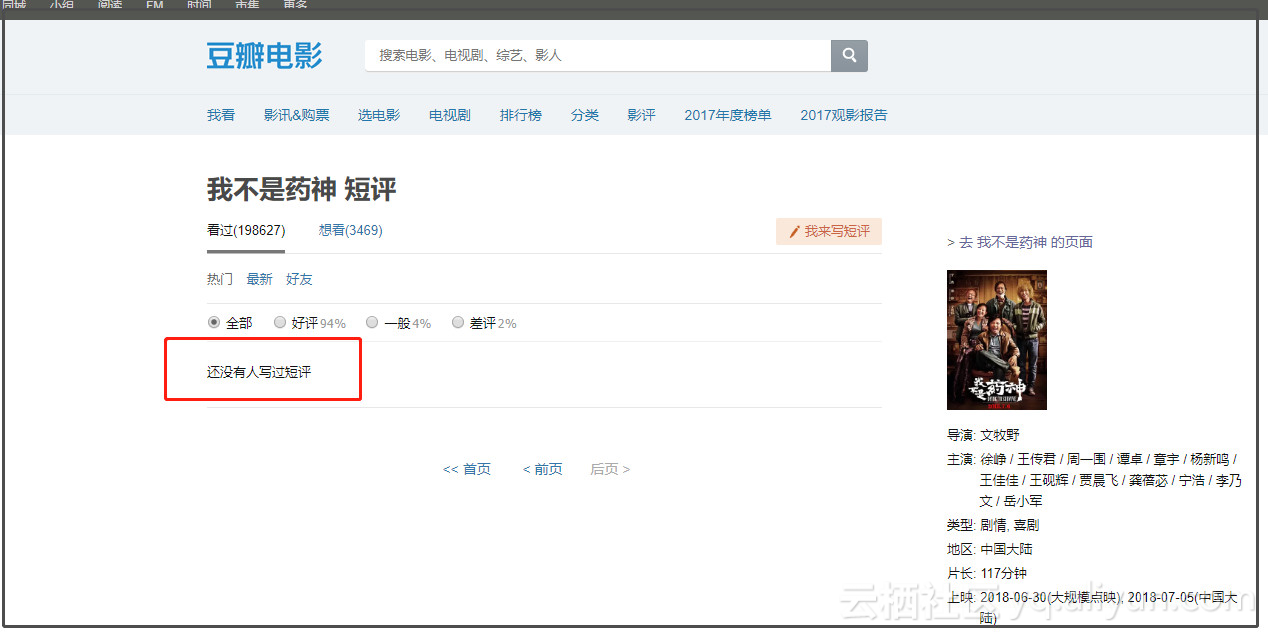4.数据清洗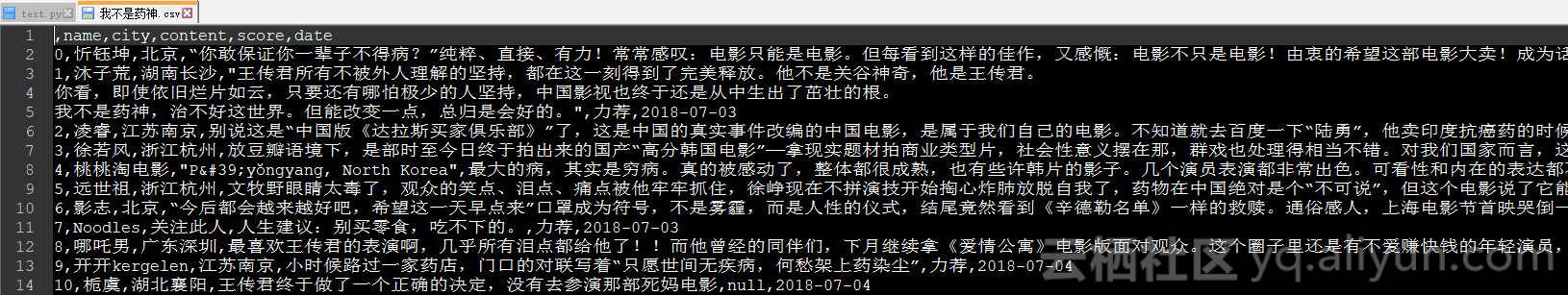4.1 城市信息清洗

pyecharts尚不支持的城市，目前支持的城市列表可以看到Github相关链接：
https://github.com/pyecharts/pyecharts/blob/master/pyecharts/datasets/city_coordinates.json
step1: 过滤筛选中文字

line = str.strip()
p2 = re.compile('[^\u4e00-\u9fa5]')  # 中文的编码范围是：\u4e00到\u9fa5
zh = " ".join(p2.split(line)).strip()
zh = ",".join(zh.split())
str = re.sub("[A-Za-z0-9!！，%,。]", "", zh)


step2: 匹配pyecharts支持的城市列表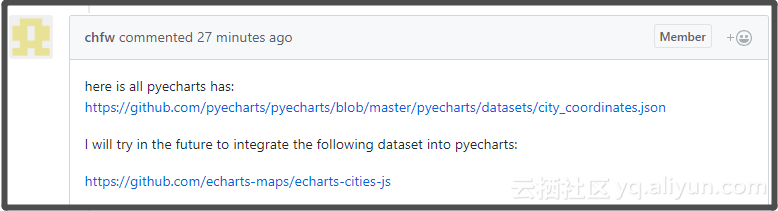· 两个字：北京
· 三个字：攀枝花
· 四个字：山东烟台
· 五个字：四川攀枝花
· 六个字：黑龙江哈尔滨

d = pd.read_csv(csv_file, engine='python', encoding='utf-8')
for i in d['city'].dropna():       # 过滤掉空的城市
i = translate(i)  # 只保留中文
if len(i)>1 and len(i)<5:  # 如果名称个数2~4，先判断是否在字典里
if i in fth:
citys.append(i)
else:
i = i[-2:]  # 取城市名称后两个，去掉省份
if i in fth:
citys.append(i)
else:
continue
if len(i) > 4:
if i in fth:   # 如果名称个数>2，先判断是否在字典里
citys.append(i)
if i[-5:] in fth:
citys.append(i[-5:])
continue
if i[-4:] in fth:
citys.append(i[-4:])
continue
if i[-3:] in fth:
citys.append(i[-3:])
else:
continue
result = {}
while '' in citys:
citys.remove('')  # 去掉字符串中的空值
print("城市总数量为：",len(citys))
for i in set(citys):
result[i] = citys.count(i)
return result


while True:   # 二次筛选，和pyecharts支持的城市库进行匹配，如果报错则删除该城市对应的统计
try:
attr, val = geo.cast(info)
geo.add("", attr, val, visual_range=[0, 300], visual_text_color="#fff", is_geo_effect_show=False,
is_piecewise=True, visual_split_number=6, symbol_size=15, is_visualmap=True)
except ValueError as e:
e = str(e)
e = e.split("No coordinate is specified for ")  # 获取不支持的城市名称
info.pop(e)
else:
break


5.基于snownlp的情感分析

snownlp主要可以进行中文分词（算法是Character-Based Generative Model）、词性标注（原理是TnT、3-gram 隐马）、情感分析（官网木有介绍原理，但是指明购物类的评论的准确率较高，其实是因为它的语料库主要是购物方面的，可以自己构建相关领域语料库，替换原来的，准确率也挺不错的）、文本分类（原理是朴素贝叶斯）、转换拼音、繁体转简体、提取文本关键词（原理是TextRank）、提取摘要（原理是TextRank）、分割句子、文本相似（原理是BM25）。

attr, val = [], []
info = count_sentiment(csv_file)
info = sorted(info.items(), key=lambda x: x, reverse=False)  # dict的排序方法
for each in info[:-1]:
attr.append(each)
val.append(each)
line = Line(csv_file+":影评情感分析")
line.render(csv_file+"_情感分析曲线图.html")
6. 数据可视化与解读


6.0 文本读取

step1 获取文件绝对路径
step2 转换路径中的为\

path = os.path.abspath(os.curdir)
csv_file = path+ "\\" + csv_file +".csv"
csv_file = csv_file.replace('\\', '\\\\')


6.1评论来源城市分析

except ValueError as e:
e = str(e)
e = e.split("No coordinate is specified for ")  # 获取不支持的城市名称
info.pop(e)


for key in info:
attr.append(key)
val.append(info[key])


geo1 = Geo("", "评论城市分布", title_pos="center", width=1200, height=600,
background_color='#404a59', title_color="#fff")
geo1.add("", attr, val, visual_range=[0, 300], visual_text_color="#fff", is_geo_effect_show=False,
is_piecewise=True, visual_split_number=10, symbol_size=15, is_visualmap=True, is_more_utils=True)
#geo1.render(csv_file + "_城市dotmap.html")
geo2 = Geo("", "评论来源热力图",title_pos="center", width=1200,height=600, background_color='#404a59', title_color="#fff",)
geo2.add("", attr, val, type="heatmap", is_visualmap=True, visual_range=[0, 50],visual_text_color='#fff', is_more_utils=True)
#geo2.render(csv_file+"_城市heatmap.html")  # 取CSV文件名的前8位数
bar = Bar("", "评论来源排行", title_pos="center", width=1200, height=600 )
bar.add("", attr, val, is_visualmap=True, visual_range=[0, 100], visual_text_color='#fff',mark_point=["average"],mark_line=["average"],
is_more_utils=True, is_label_show=True, is_datazoom_show=True, xaxis_rotate=45)
#bar.render(csv_file+"_城市评论bar.html")  # 取CSV文件名的前8位数
pie = Pie("", "评论来源饼图", title_pos="right", width=1200, height=600)
#pie.render(csv_file + "_城市评论Pie.html")  # 取CSV文件名的前8位数
page.render(csv_file + "_城市评论分析汇总.html")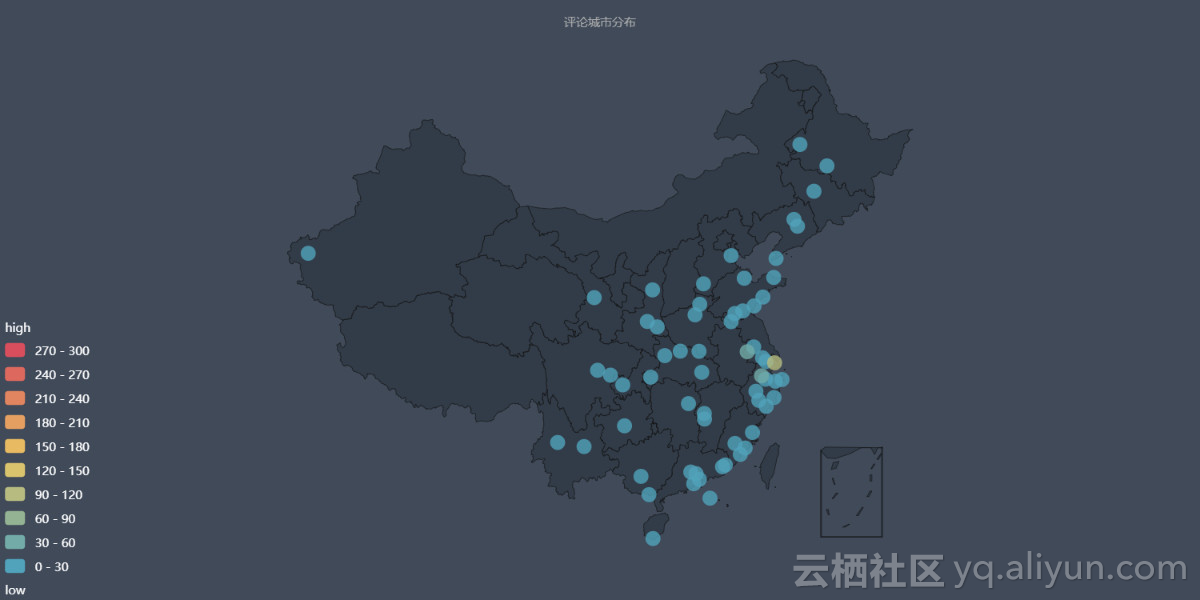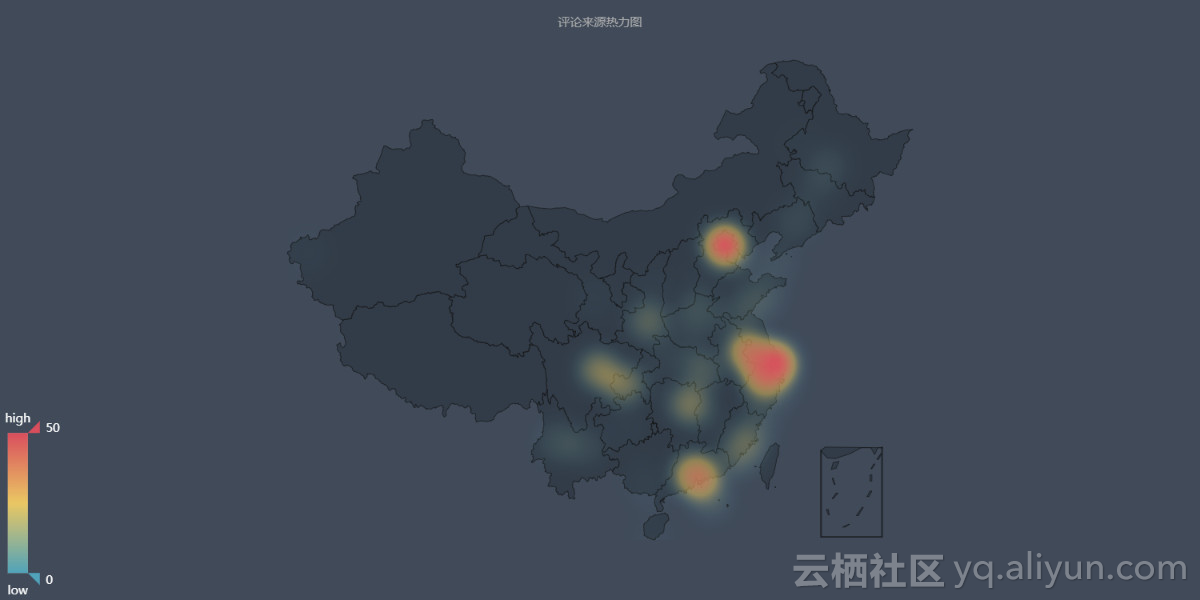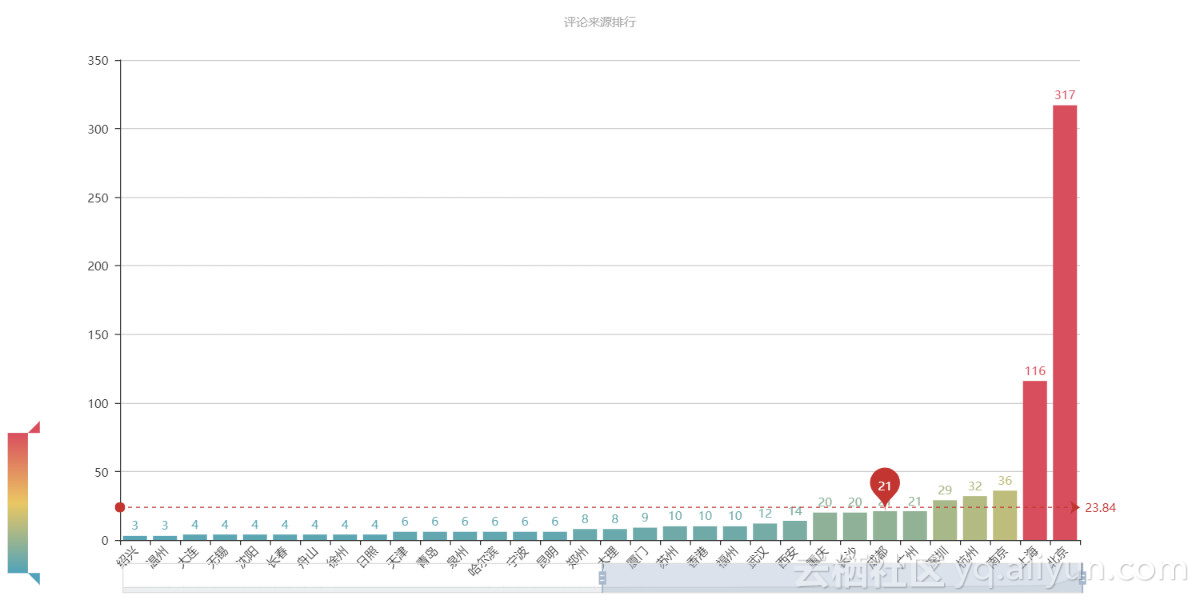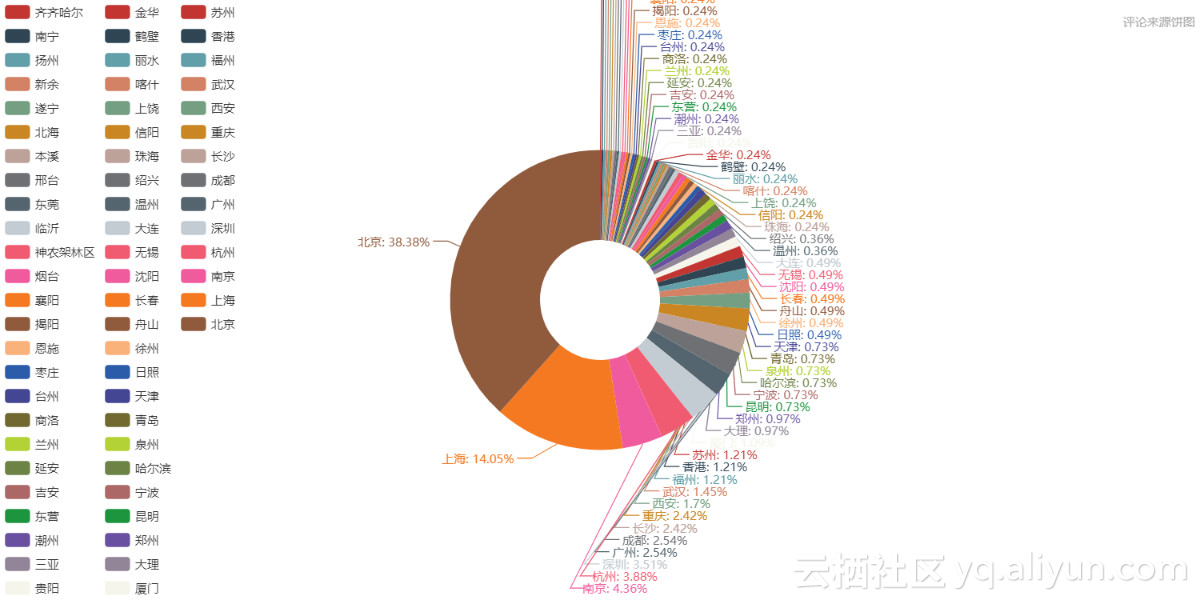6.2 评论情感分析

0.5以下为负面情绪，0.5以上为正面情绪，因为电影好评太多，为了图形的合理性（让低数值的统计量也能在图中较明显的展示），把评论接近1的去掉了。当然按理说情绪正面性到1的应该很少，出现这种结果的原因我觉得是语料库的锅。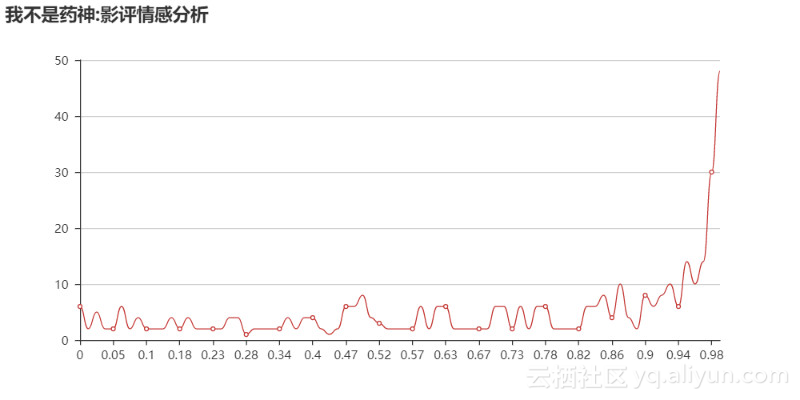6.3 电影评分走势分析

info_new.sort_values('date', inplace=True)

creat_df = pd.DataFrame(columns = ['score', 'date', 'votes']) # 创建空的dataframe
for i in list(info_new['date']):
location = info_new[(info_new.date==i)&(info_new.score=="力荐")].index.tolist()
if location == []:
creat_df.loc[mark] = ["力荐", i, 0]
mark += 1
location = info_new[(info_new.date==i)&(info_new.score=="推荐")].index.tolist()
if location == []:
creat_df.loc[mark] = ["推荐", i, 0]
mark += 1
location = info_new[(info_new.date==i)&(info_new.score=="还行")].index.tolist()
if location == []:
creat_df.loc[mark] = ["还行", i, 0]
mark += 1
location = info_new[(info_new.date==i)&(info_new.score=="较差")].index.tolist()
if location == []:
creat_df.loc[mark] = ["较差", i, 0]
mark += 1
location = info_new[(info_new.date==i)&(info_new.score=="很差")].index.tolist()
if location == []:
creat_df.loc[mark] = ["很差", i, 0]
mark += 1
info_new = info_new.append(creat_df.drop_duplicates(), ignore_index=True)


info_new = info_new.append(creat_df.drop_duplicates(), ignore_index=True)


for index, row in info_new.iterrows():   # 第二种遍历df的方法


for indexs in d.index:  # 一种遍历df行的方法
d.loc[indexs].values[:])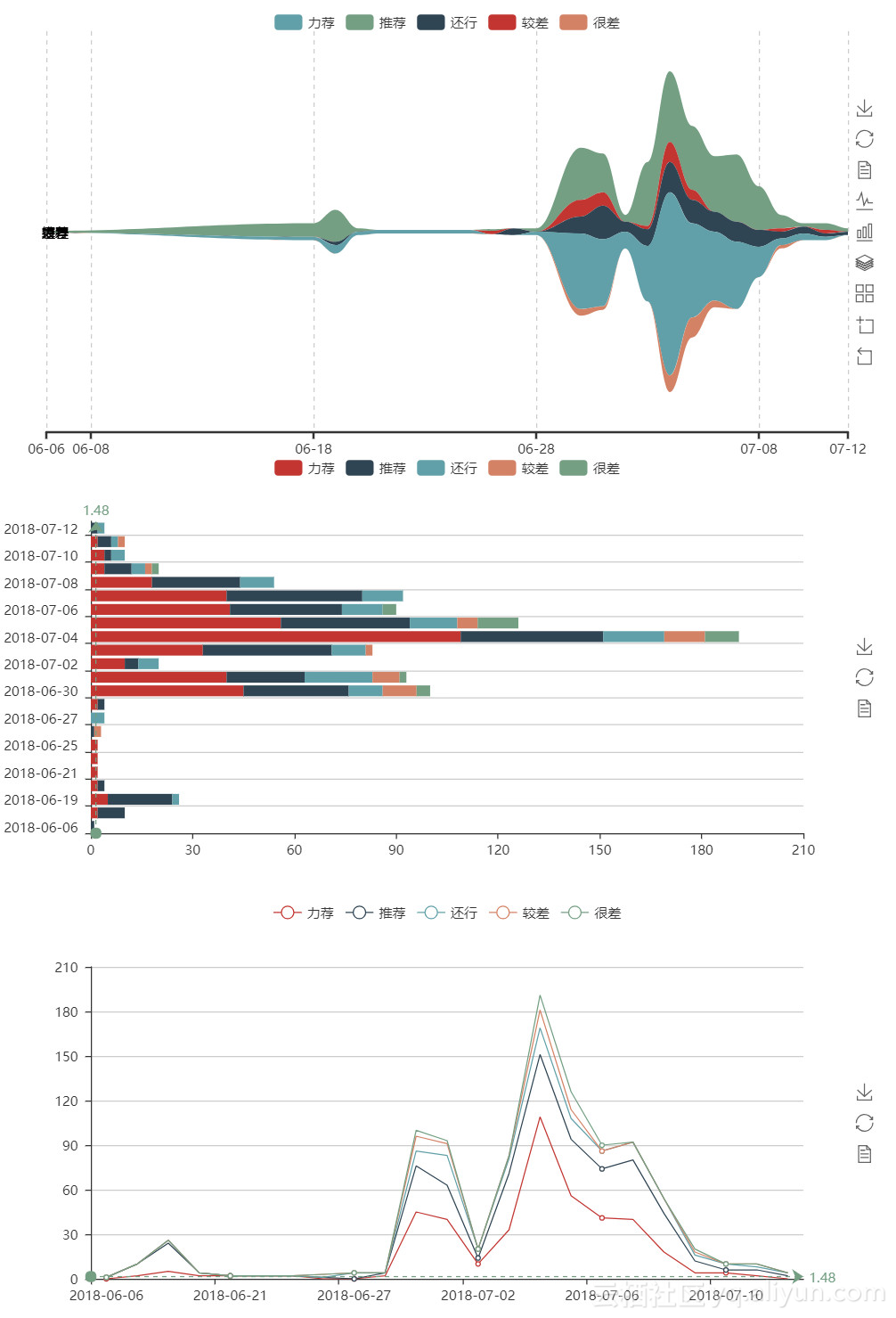6.4 影评词云图《邪不压正》词云图

一、治脚吗？不治。治治吧。不治。治治。《邪不压正》《我不是药神》词云图

7.总结

pyecharts画图挺别致的。

+ 关注